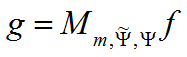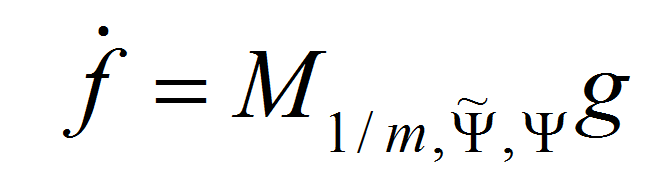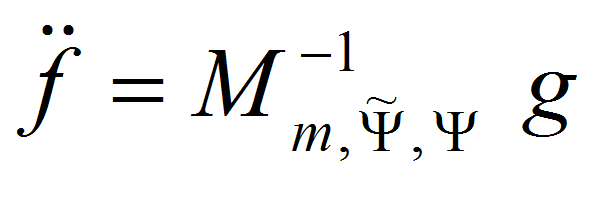This page provides resources for the visualizations and the algorithms in the research manuscript:

# "Representation of the inverse of a frame multiplier"Peter Balazs and Diana T. Stoeva

## Abstract

Certain mathematical objects appear in a lot of scientific disciplines, like physics, signal processing and, naturally, mathematics. In a general setting they can be described as frame multipliers, consisting of analysis, multiplication by a fixed sequence (called the symbol), and synthesis. They are not only interesting mathematical objects, but also important for applications, for example for the realization of time-varying filters. In this paper we show a surprising result about the inverse of such operators, if existing, as well as new results about a core concept of frame theory, dual frames. We show that for semi-normalized symbols, the inverse of any invertible frame multiplier can always be represented as a frame multiplier with dual frames and reciprocal symbol. Furthermore, one of those dual frames is uniquely defined and the other one can be arbitrarily chosen. We investigate sufficient conditions for the special case, when both dual frames can be chosen to be the canonical duals. In connection to the above, we show that the set of dual frames determines a frame uniquely. Furthermore, for a given frame, the union of all coefficients of its dual frames is dense in l2. We investigate invertible Gabor multipliers; we show that the inverse of every invertible lattice-invariant operator (in particular, every invertible Gabor frame multiplier with a constant symbol (1)) can be represented as a Gabor frame multiplier with a constant symbol (1). Finally we give a numerical example for the invertibility of multipliers in the Gabor case.

## Figures:

### Figure 1 in the manuscript (an illustrative example to visualize actions of a multiplier on a musical signal)

In Figure 1 we show a visualization of a multiplier Mm,Φ,Ψ in the time-frequency plane. We consider a music signal f and the action of a multiplier Mm,Φ,Ψ on f. For f we use a 2 seconds long excerpt of the "Jump" from Van Halen (click here to listen the signal). For a time-frequency representation of the musical signal f (TOP LEFT) we use a 'painless' Gabor frame Ψ (a 80 ms Hanning window with 12,5% overlap). By manual estimation, we determine the symbol m that should describe the time-frequency region of the singer's voice. This region is then multiplied by 0.01, the rest by 1 (TOP RIGHT) (see the symbol here). Finally, we show a time-frequency representation of the modified signal Mm,Φ,Ψf (BOTTOM). To listen the modified signal,(TOP LEFT) The time-frequency representation of the music signal f (TOP RIGHT) The symbol m, found by a (manual) estimation of the time-frequency region of the singer's voice. (BOTTOM) Time-Frequency representation of Mm,Φ,Ψf.

### Figure 2 in the manuscript (numerical visualization of inversion of multipliers)

Here we use the same signal f and the same multiplier Mm,Φ,Ψ as in Figure 1. Note that all the elements of the symbol m fulfill mn,k∊{1,10-2}. Since m is semi-normalized, the multiplier M is analytically invertible . However, the operator is badly conditioned, the condition number is around 99. The signal f is approximately 2 seconds long, using a sampling rate of 44100. Thus, the signal is a 128148-dimensional vector.

Starting fromwe compare two approaches numerically:

• a 'naive' inversion<>(corresponding to the approach raised in Question [Q2] in the manuscript)

• the 'iterative' inversionClearly, the naive approach has strong artifacts. The error is especially big at the boundaries of the constant region of the symbols. The chosen atoms are well localized in time-frequency, so that within the interior of the constant regions, this inversion works well. This could be expected as we have shown in the manuscript that constant symbols allow this kind of inversion for equivalent frames.

The iterative inversion worked well with an error of 3%. This could, naturally, be decreased by investing more calculation time. But also in the chosen setting for the iterative inversion (100 iterations in iframemul ) no difference can be seen in the time-frequency representation, as well as no audible difference can be detected.(TOP LEFT) The time-frequency representation of the result of the 'naive' inversion. (TOP RIGHT) The time-frequency representation of the error of the 'naive' inversion, i.e.. (BOTTOM LEFT) The time-frequency representation of the iterative inversion. (BOTTOM RIGHT) The time-frequency representation of  the error of the iterative inversion.

## Algorithms:

The above visualizations are done using algorithms in the Matlab/Octave toolbox Linear Time-Frequency Analysis (LTFAT)  (version 1.4.0 and above). In order to run the script, provided below, first you need to install the the Matlab/Octave toolbox LTFAT, freely available at Sourceforge.

The Matlab script for producing Figures 1 and 2 is available for download here. To run the scrip, you need to have the following two files in the same folder:

Running the script, the output is the Figures 1 and 2.

## References:

•  D. T. Stoeva and P. Balazs, "Invertibility of multipliers", Applied and Computational Harmonic Analysis, vol. 33 (2012), 292-299.
•  P. Søndergaard, B. Torrésani, and P. Balazs,"The linear time frequency analysis toolbox", International Journal of Wavelets, Multiresolution and Information Processing, vol. 10, no. 4, pp. 1250032, 2012.